# Module 8: Loops

## Lesson Objectives

After reading this lesson, you should be able to:

• apply knowledge of loop fundamentals to create nested loops,

• write programs using nested loops,

• use programming flags in loops.

## What is a nested loop?

Nested loops are just what they sound like: a loop within a loop. There are no programming limitations on how many nested loops you can create. By definition, you need a minimum of two loops to have nested loops: one parent and one nested loop. For example, a for loop nested inside another for loop as seen in Figure 1.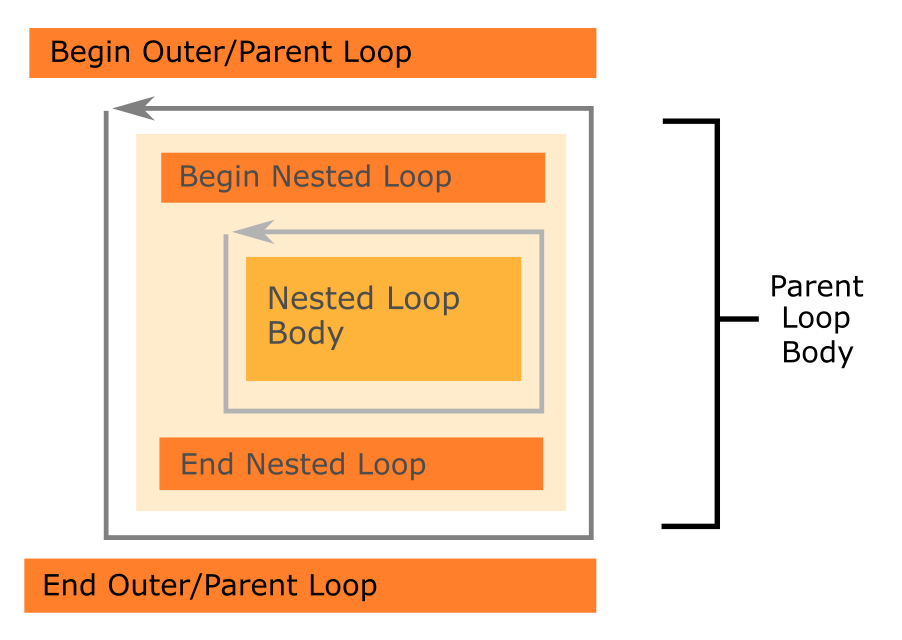Figure 1: Depicts nested loops where one nested (or inner) loop is contained inside the body of the parent (or outer) loop.

## How do nested loops work?

We will go into more examples of nested loop applications in the following lessons. For now, imagine you want to display each element of a vector individually. You will need one for loop to “look” through all of those elements. Now, suppose you want to display all the elements of a matrix. Remember, you can think of a matrix as a collection of vectors. You will need to run a for loop for each vector contained in the matrix! That is, you will need one for loop for each column of the matrix and one for loop for each row of the matrix. So, the block of code you want to repeat contains a loop.

### Example 1

Show each element of any given matrix one element at a time in the Command Window. Test the program with the matrix $$A$$.

$A= \begin{bmatrix} 7 & 11 \\ 13 & 19 \\ 23 & 31 \end{bmatrix}$

Solution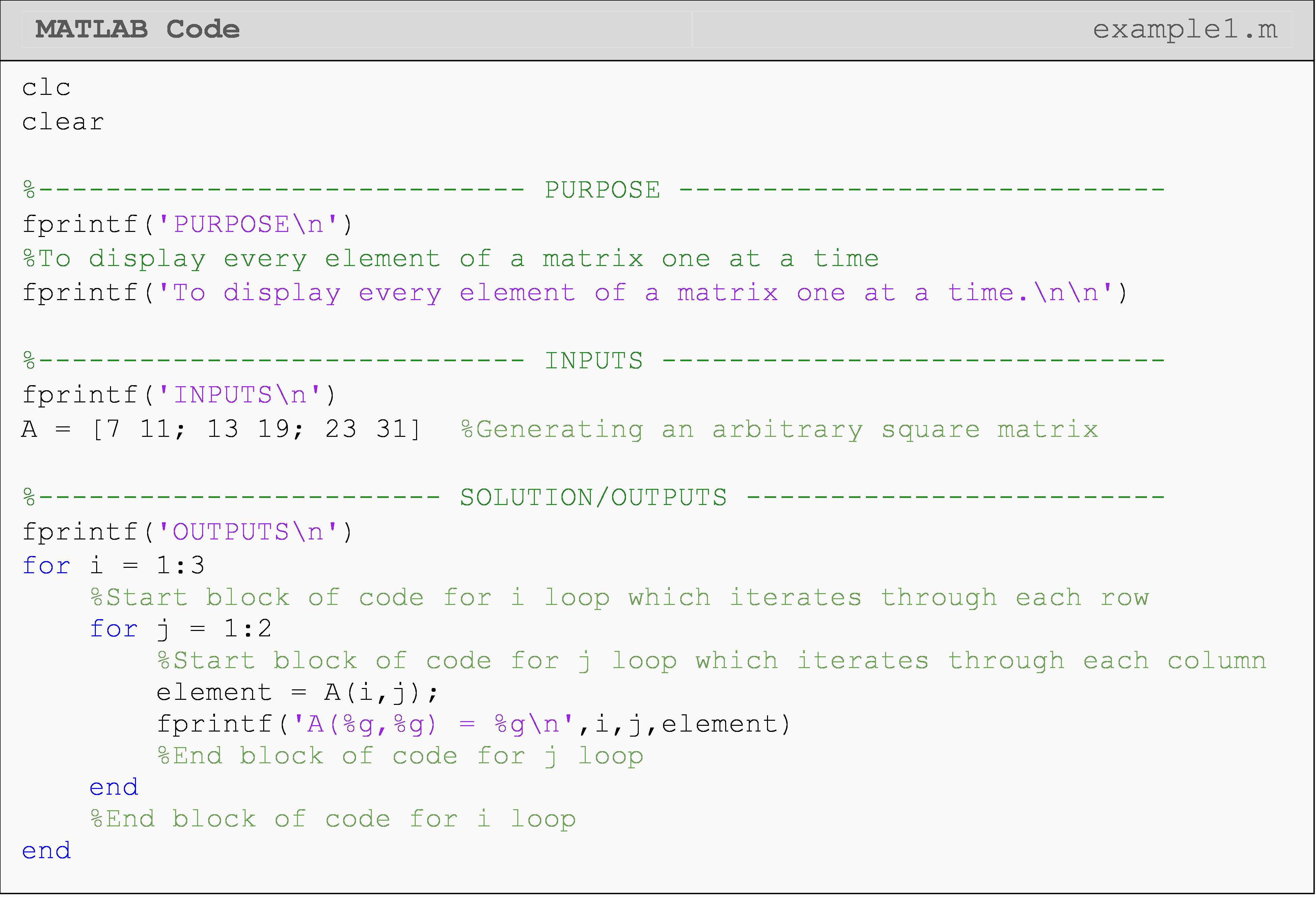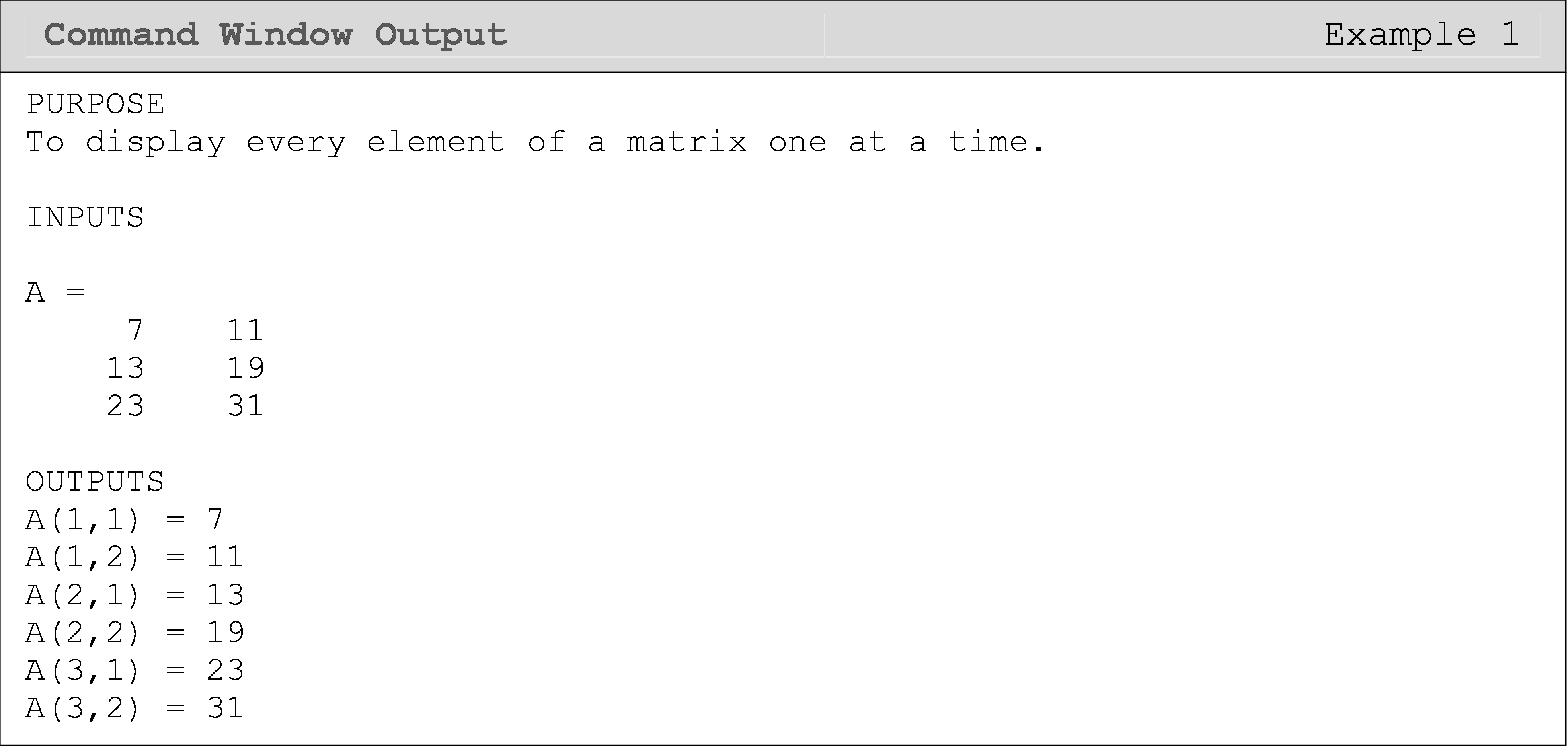## How do loop mechanics apply to nested loops?

Loop mechanics refers to how loops function fundamentally. For example, how a loop runs a block of code multiple times or how variables are referenced from the previous iteration values. The code will run the entire nested loop before going to the next iteration of the parent loop. You can see this general property of loops exhibited in Example 2. Notice the outer loop only iterates after its block of code executes, which includes the inner loop.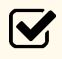Important Note: The code will run the entire nested loop before going to the next iteration of the parent loop.

### Example 2

Demonstrate the mechanics of nested loops by displaying the values of the loop iterators each time either loop iterates.

Solution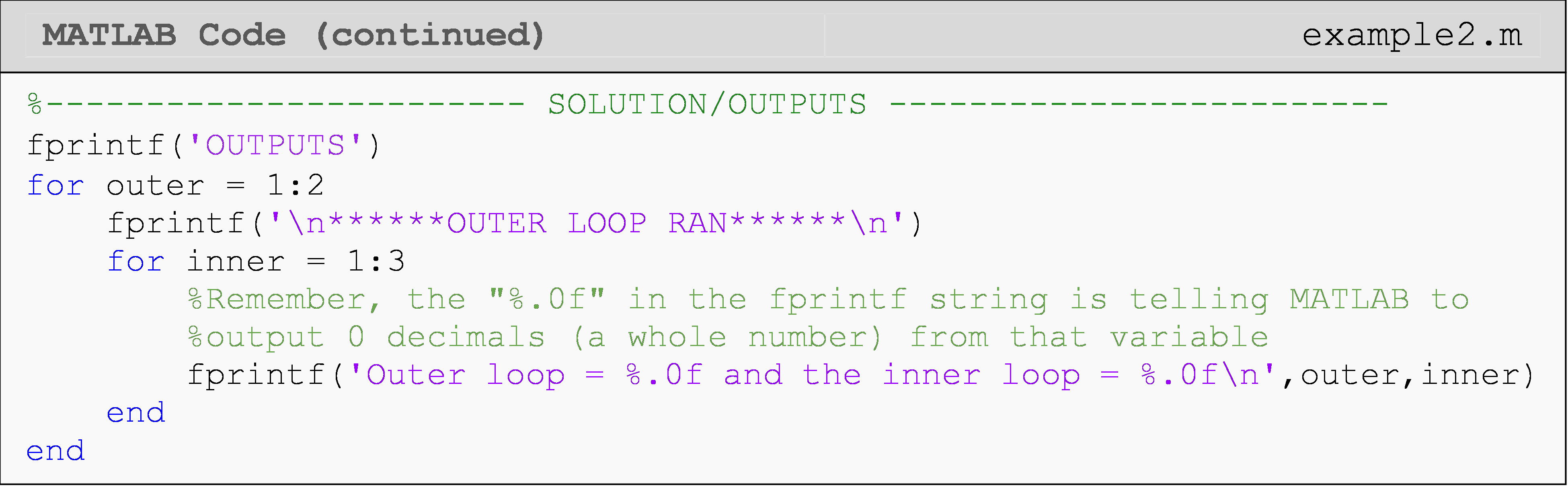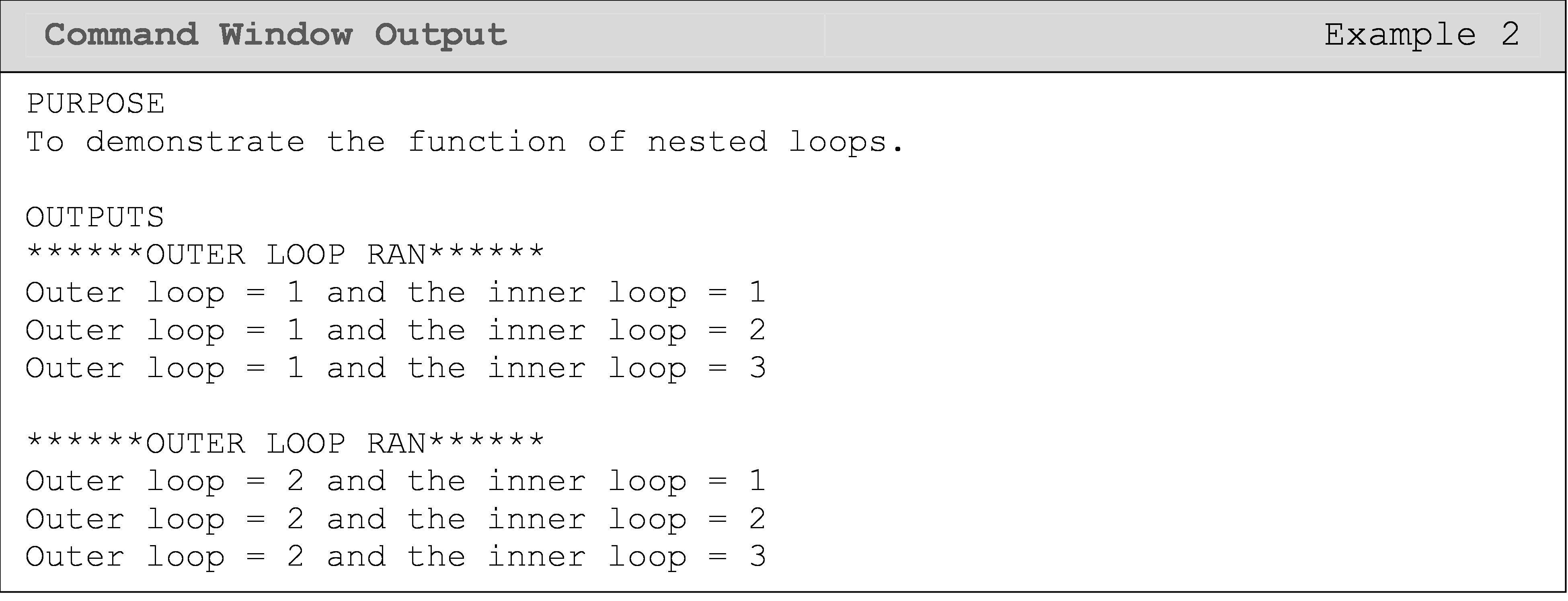We can see from both the solution and its result (in the Command Window output) that the program must complete a total of $$2 \times 3 = 6$$ repetitions.

You can mix and match your nested loops. For example, you can do for-for, while-for, for-while, etc. It just depends on what you need in your solution to a problem. Of course, the general principle of the inner loop being complete before the outer loop iterates remains true as demonstrated in Example 4.

## What is a “flag”?

A programming trick often used in loops (especially nested loops) is something called flags. Flags are not a special data type; they are simply a way of signaling in the program. It is kind of like shooting up a flare or raising a physical flag on a mailbox that another part of the program “sees”. We will use flags in Examples 3, 4, and 5.

Typically, one would include the phrase “flag” in the variable name to differentiate it from other variables. It is also good practice to write the different states of the variable when it is first defined. For example, we could define a variable named stopFlag and write a comment stating “true means the operation should end”.

## When should I use programming flags?

A flag is typically a numeric or Boolean value (Example 3 uses a Boolean flag). If you need a refresher on Boolean, review Lesson 5.1.Important Note: You can name the flag variable anything just like any other variable.

### Example 3

Search any given vector to find the first instance of a given number (if it contains the number). Display a message in the Command Window the first instance that value is found and where its location is within the vector. Use the vector vec = [1 3 2 9 4 10 4] and look for the number 4 to test the program.

Solution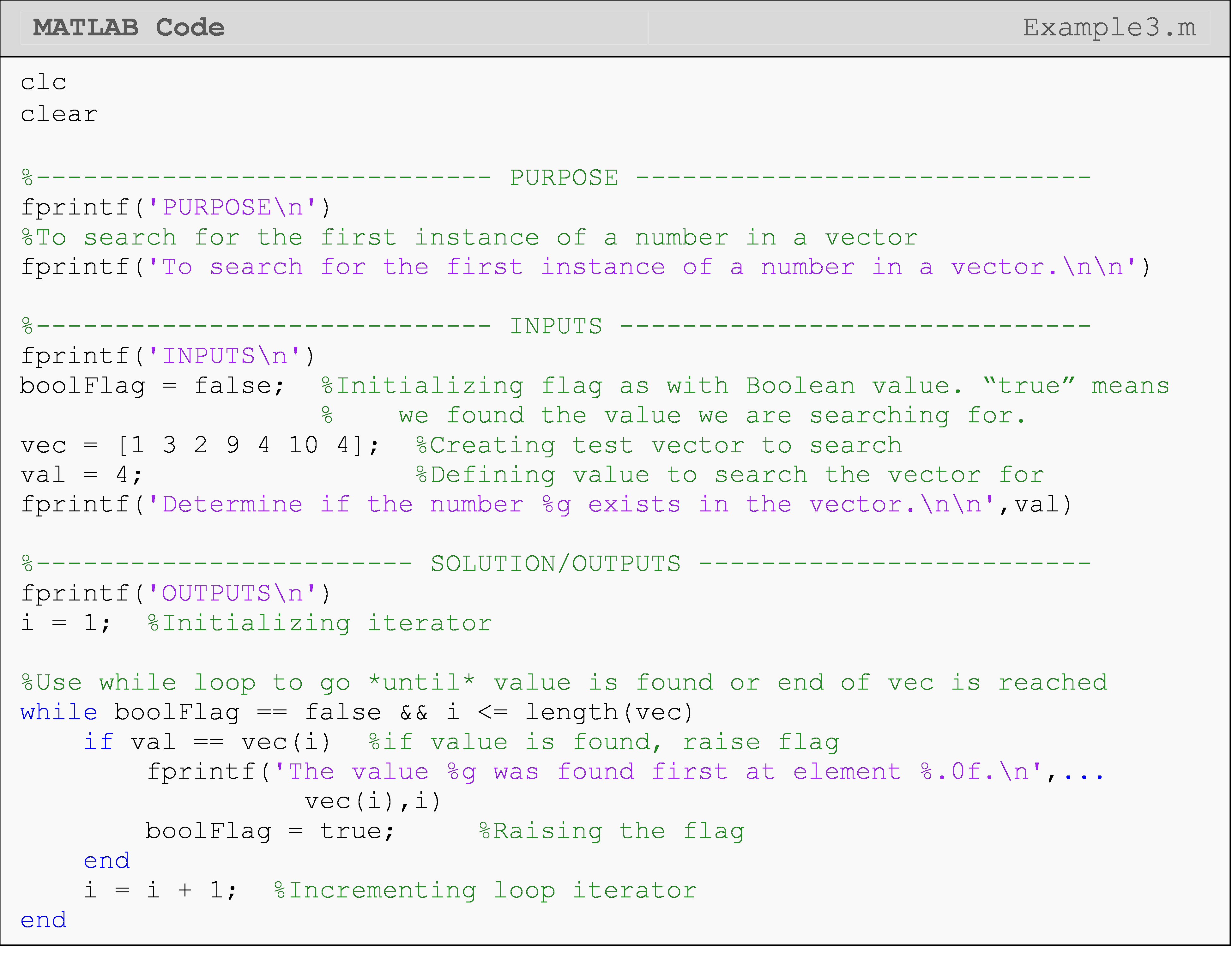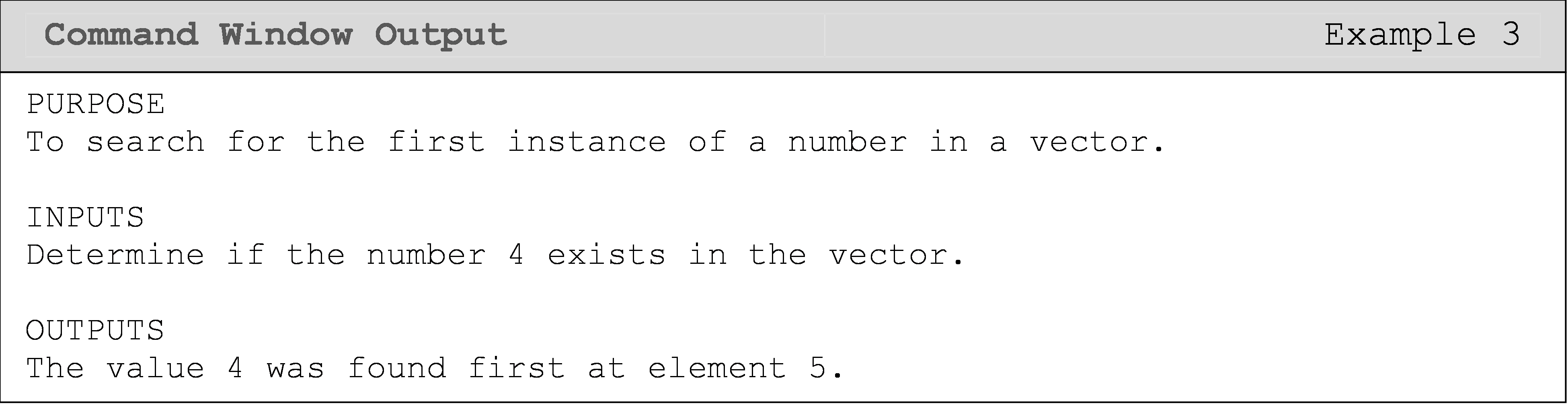Note that this solution stops if one instance of the desired value is found. You can see this since there are two instances of 4 in the given vector, but only one is displayed. The solution can be modified to find all the instances of the desired value in an array.

In Examples 3, 4, and 5, Boolean variables are used as programming flags. They are binary cases that can only either be true or false. This is the most common use case in programming as it makes things very clear at a glance (e.g., isEmpty = false, so we know the thing is not empty). However, there are some cases that have more than two states we would like to represent. In these cases, we can use numbers or strings as the values for the flag.

You are tasked with developing a program in MATLAB that generates six random unique integers in the 1-53 range and stores each integer in a vector called luckySix. Store each integer in the order in which it was picked (i.e., the first number generated is stored as the first element of vector luckySix and so on). The program must display these unique integers in the Command Window.

### Example 4

Solution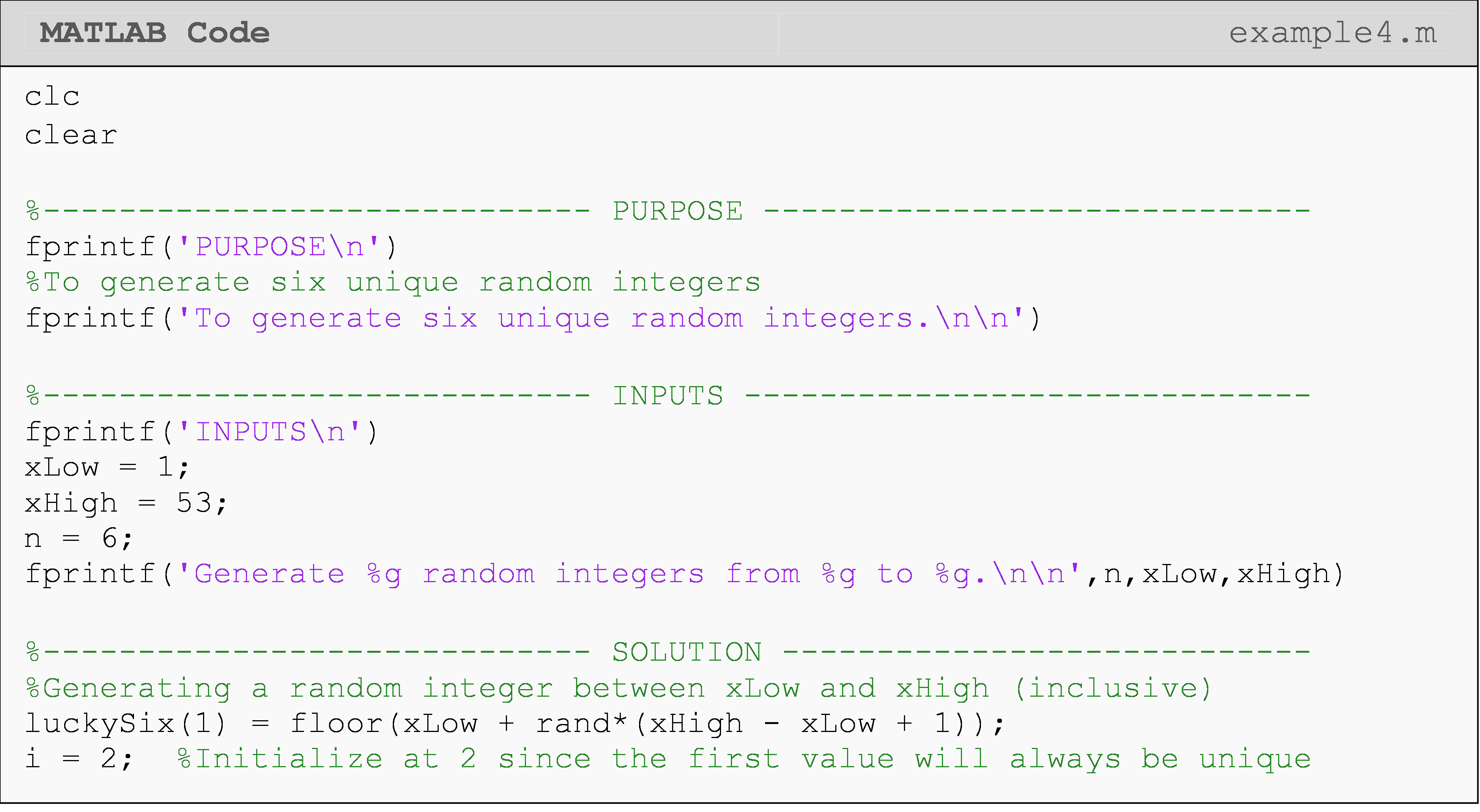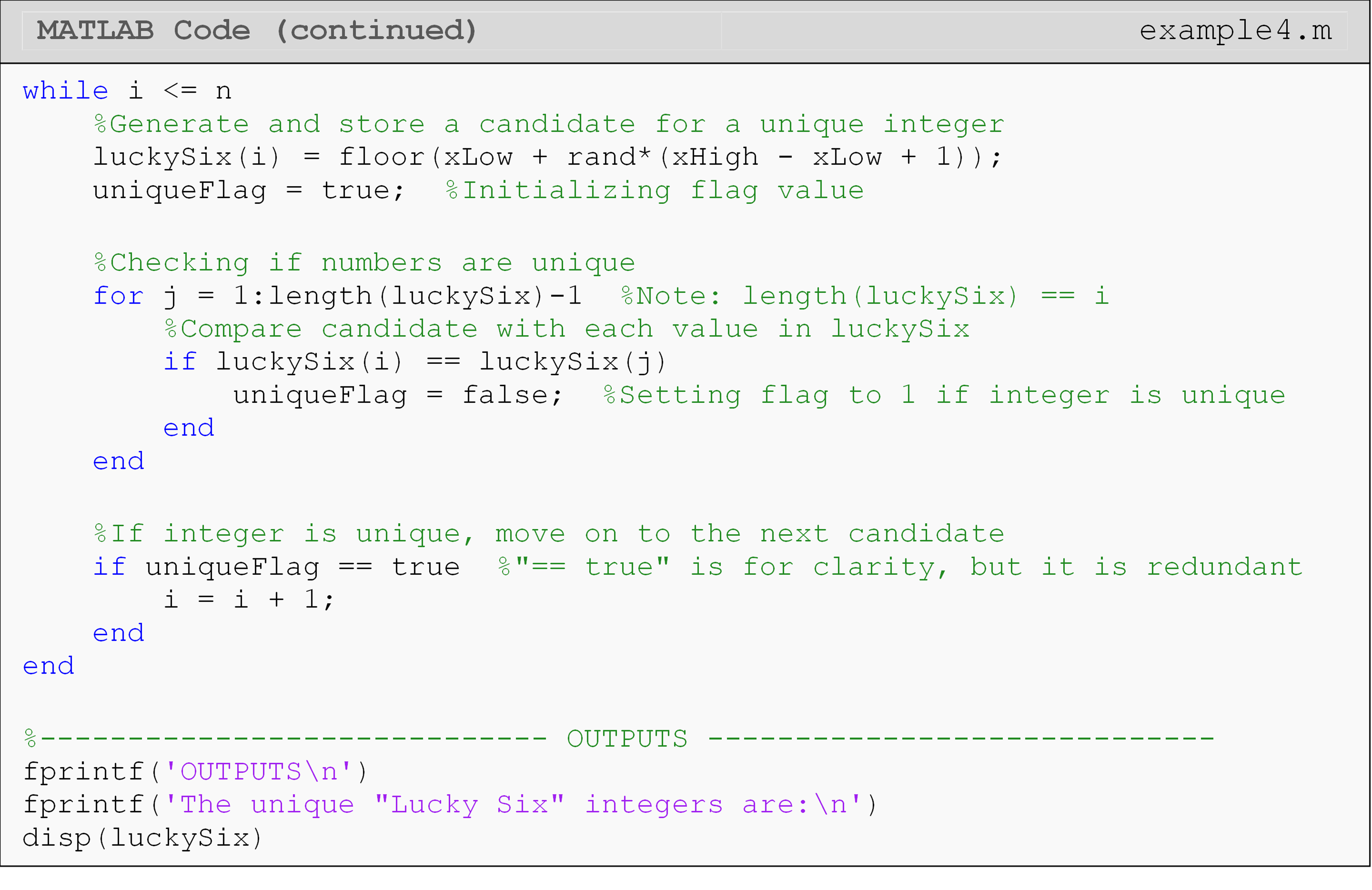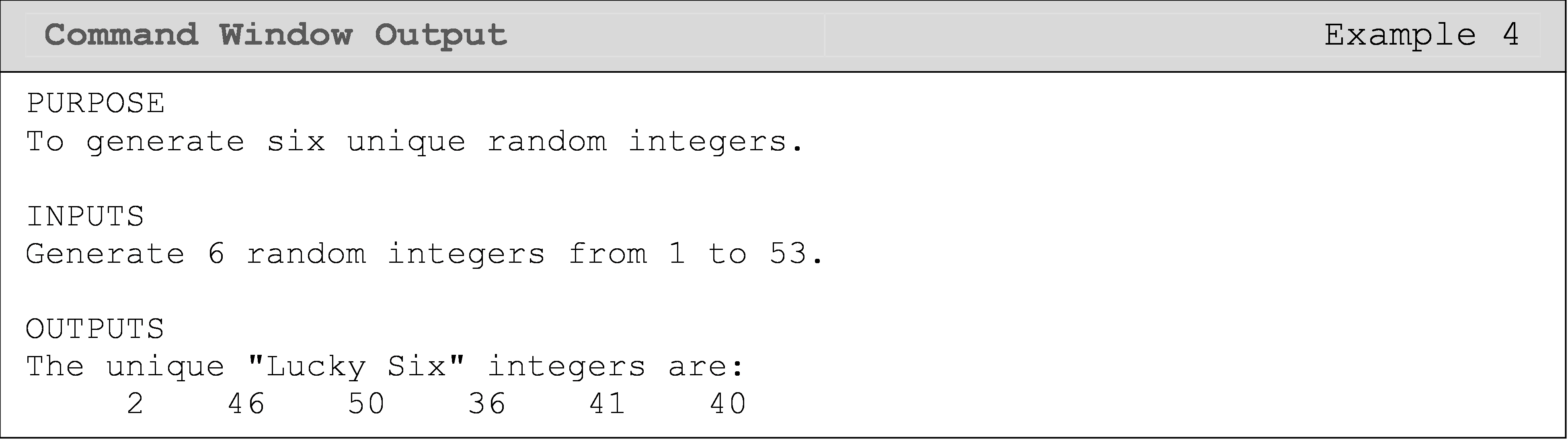One can see that two loops are required to complete this example. In this case, the inside for loop checks the current random integer against all other previous chosen integers. The while loop is used because we do not know the exact number of repetitions it would take to pick six unique integers.

Since this code relies on the rand() function, you should expect to see different results from those shown below.

## How can I use break and continue in nested loops?

When using break and continue commands in nested loops (i.e., a loop within a loop), the commands only apply inside of loop it is placed in. Calling break inside a nested loop will exit that nested loop; however, it will not exit the parent loop. Example 5 shows how you can exit both loops using a flag. Note that in Example 5, the break command appears twice: once to exit the nested loop and once to exit the parent loop.Important Note: break and continue apply only to the first loop that contains them.

Make sure you review the lesson on break and continue commands (Lesson 8.3) before trying this example.

### Example 5

Demonstrate how to use the break command inside of nested loops. Once the break command has executed within the inner loop, the program should also break out of the outer loop.

SolutionAs seen in Example 5, we need to use a second break command to break out of both loops after our condition occurs. Try removing the second break command and see what happens!

To make the code a little cleaner, we can remove the explicit check of “true” or “false” with Boolean flags (variables). In Example 5 above, if stopFlag functions the same way as if stopFlag == true.

## Multiple Choice Quiz

(1). The data type of a flag variable is

(a)  char

(b)  double

(c)  logical

(d)  any of the above

(2). The purpose of a programming flag is to

(a)  be a signal in a program

(b)  make a conditional decision

(c)  repeat a body of code

(d)  find a number in a loop

(3). Complete the code to generate a random integer between 5 and 14.
number = floor(rand*(____________))+5

(a)  14 - 5 + 1

(b)  14 - 5

(c) 5 - 1

(d)  14 - 0

(4). The Command Window output of the following program is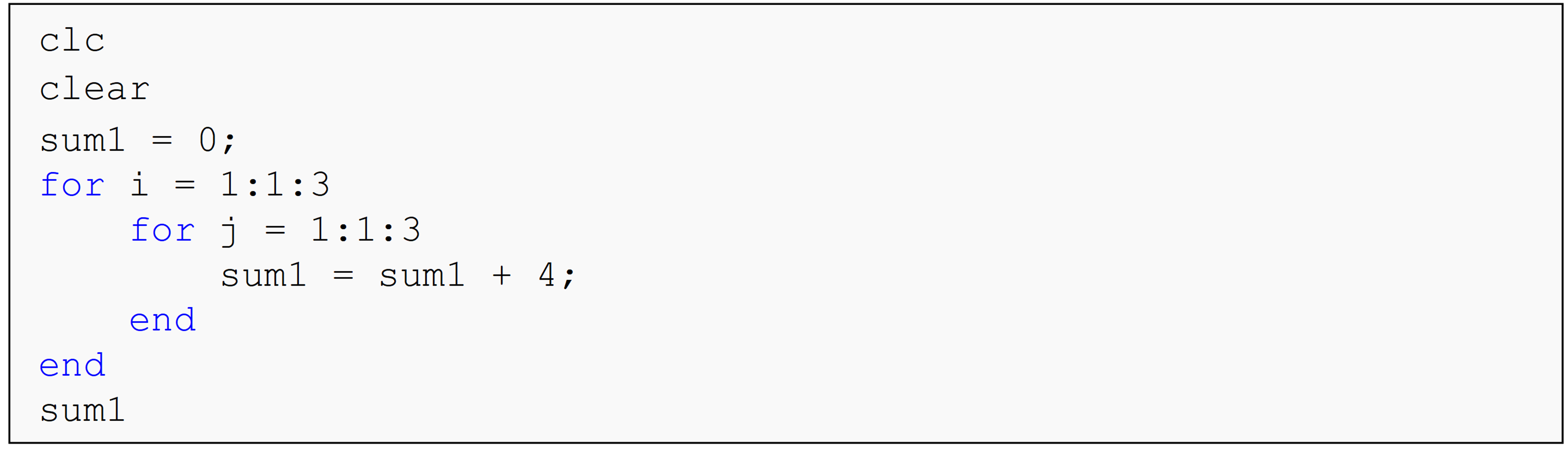(a)  sum1 = 0

(b)  sum1 = 4

(c)  sum1 = 12

(d)  sum1 = 36

(5). The Command Window output of the following program is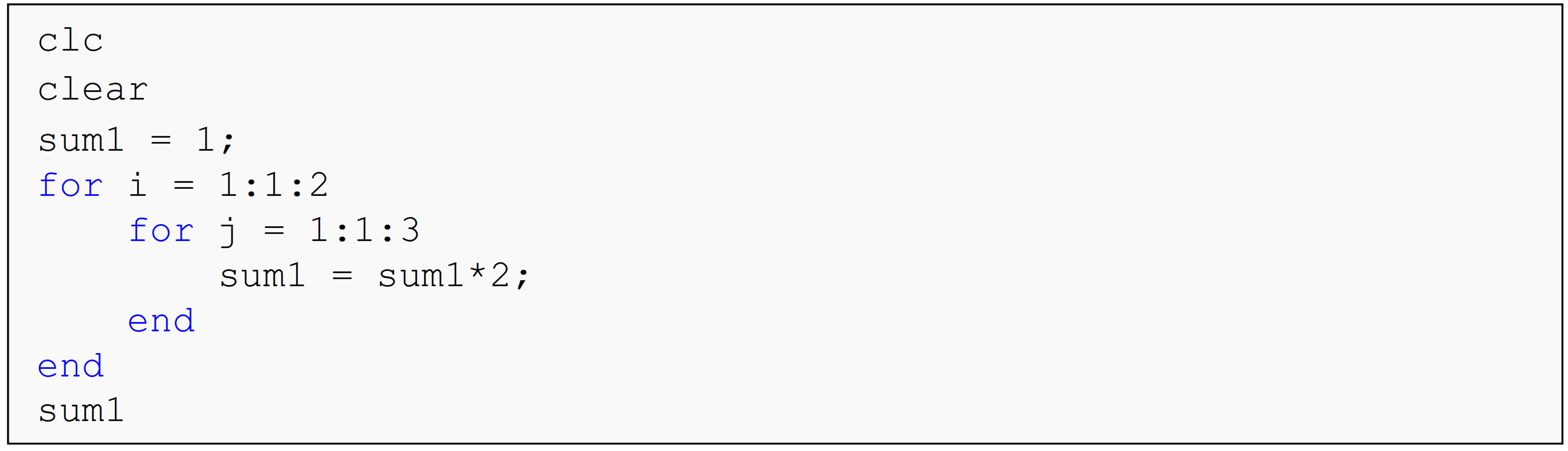(a)  sum1 = 18

(b)  sum1 = 64

(c)  sum1 = 120

(d)  sum1 = 128

(6). The Command Window output of the following program is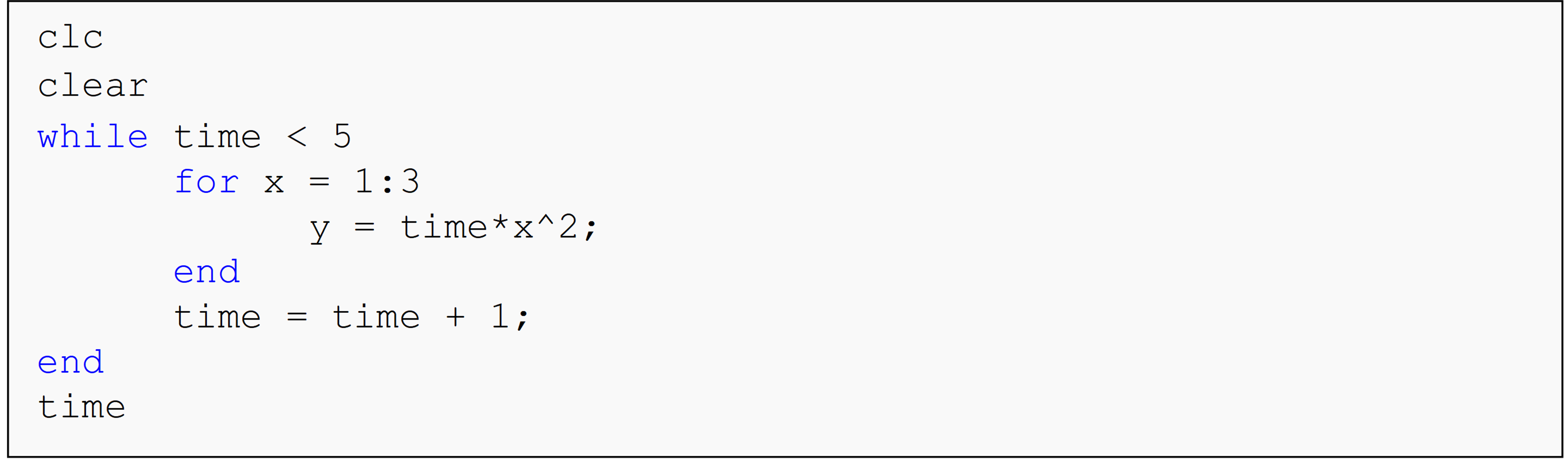(a)  time = 4

(b)  time = 5

(c)  time = 6

(d)  Undefined function or variable 'time'.

(7). The Command Window output of the following program is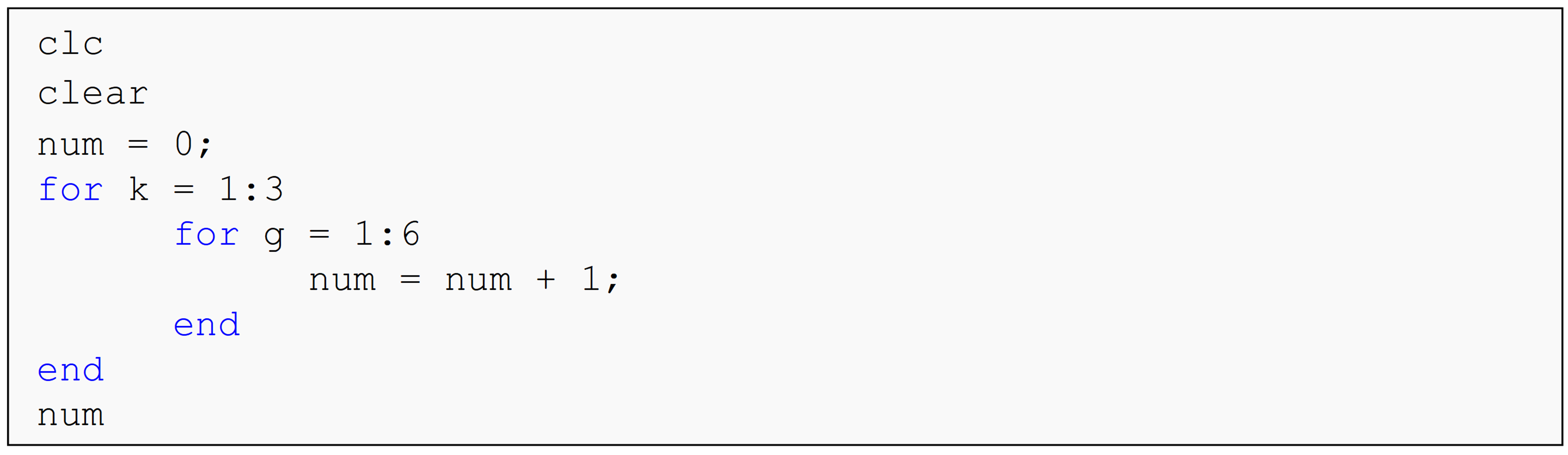(a)  num = 6

(b)  num = 3

(c)  num = 18

(d)  num = 9

(8). What is the Command Window output of the following code?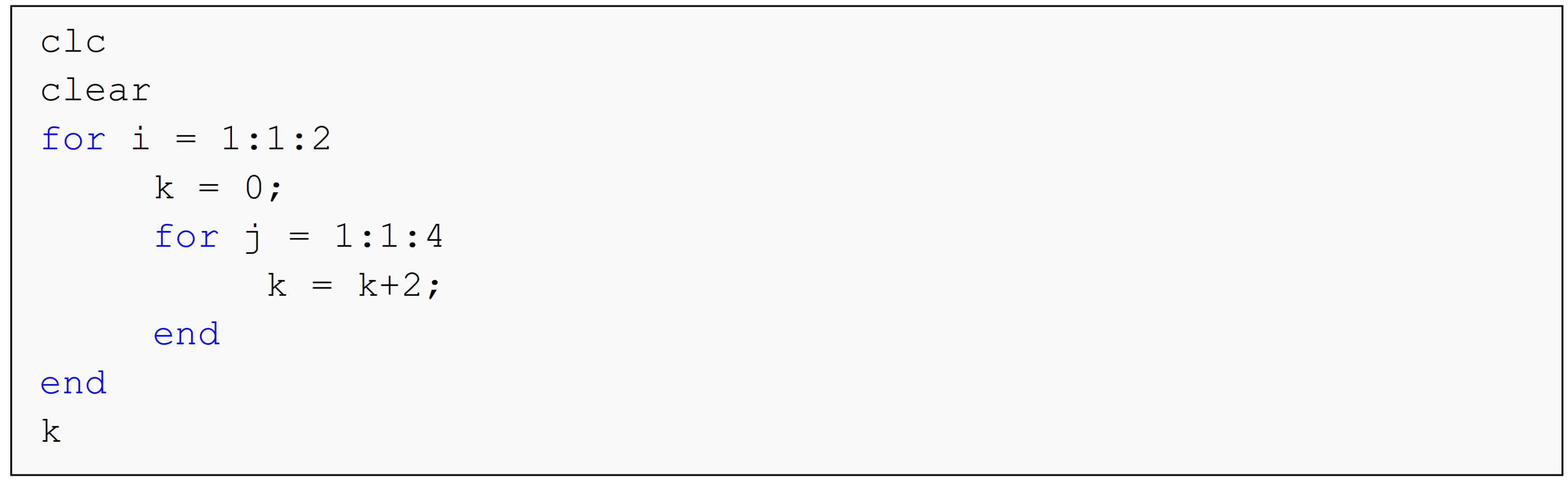(a)  k = 16

(b)  k = 8

(c)  k = 0

(d)  k = 2

(9). The value of a to make the output of the last line be s = 29 is a = ______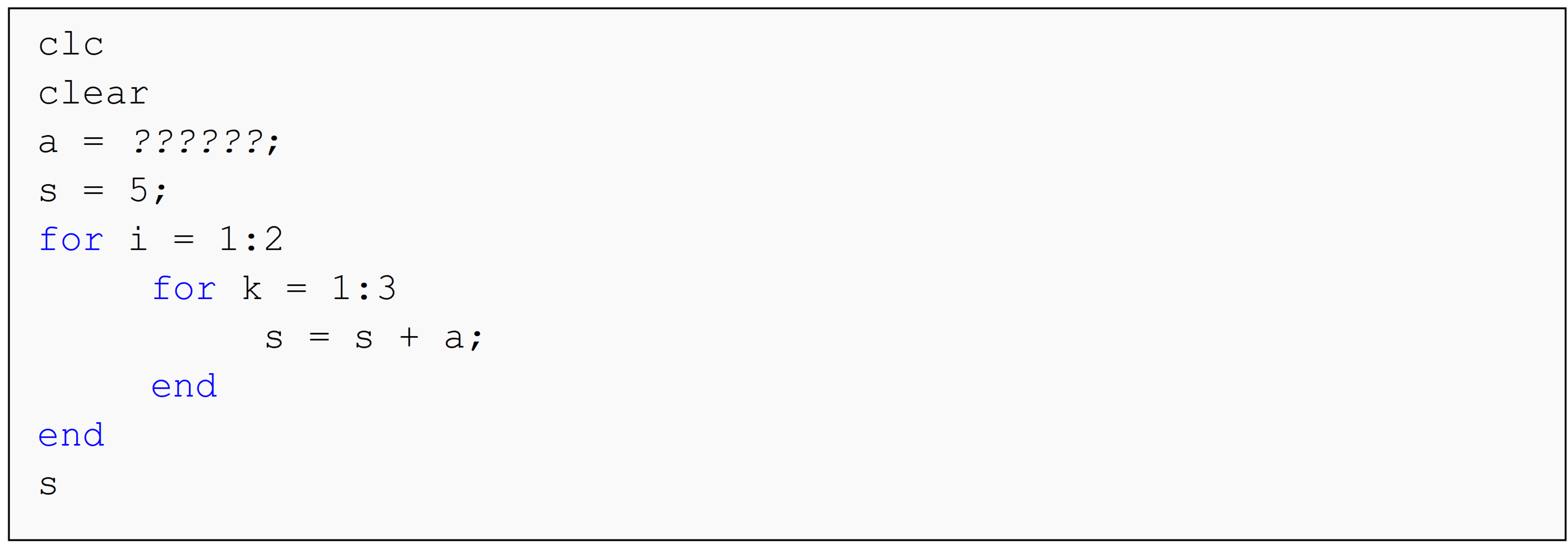(a)  4

(b)  6

(c)  24

(d)  29

## Problem Set

(1). Search any given vector to see if it contains a given number. Display a message in the Command Window each time that value is found and where its location is within the vector. Use the vector vec = [41 6 2.3 0.2 5 1 5] and look for the number 6.2 to test the program. Test again with the same vector and the number 5.

(2). Search the given vector, vec = ['M' 'A' 'T' 'L' 'A' 'B'], to see if it contains the character 'A'. Display a message in the Command Window each time that character is found and where its location is within the vector.

Hint: Use the function strcmp() to ensure the program will work for other inputs (reference Example 2 in Lesson 5.3).

(3). Write a MATLAB program that generates a vector of 8 elements where each element is a unique random integer in the domain [3, 63].

(4). Generate a matrix of pseudo-random numbers using rand(n), which will create an $$n \times n$$ matrix. Check each element of the matrix to see whether its value is at least 0.7. If a value does not meet this criterion, immediately skip to the next row of the matrix. Output each element that does not meet the criteria to the Command Window in the form: Value too low for A(i,j) = X.

(5). Generate a matrix of pseudo-random numbers using rand(n), which will create an $$n \times n$$ matrix. Check each element of the matrix to see whether its value is at least 0.25. If a value does not meet this criterion, immediately skip to the next column of the matrix. Output each element that does not meet the criteria to the Command Window in the form: Value too low for A(i,j) = X.